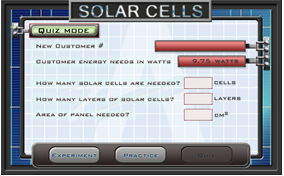# Solar CellsLarge electric company bills are hitting the wallets of people very hard. Your company has the solution: constructing and selling solar arrays that convert sunlight into electrical energy. The only problem is that it’s up to you to determine how large a solar panel each customer needs. So you’ll have to do a little testing to find out how much energy one of your solar cells produces and use some of your algebra skills to design and sell your products. Hopefully, the light will turn on in your head and you’ll make the sale.

### What is energy?

Energy is the ability to do work and exert forces on things. There are many forms of energy including kinetic energy, potential energy, thermal (or heat) energy, chemical energy, mechanical energy, nuclear energy, and electrical energy.

### What is the difference between energy transferring and energy transforming?

Energy transfer is the process of transferring energy from one object to another or from one place to another. An example of energy transfer is when you throw a rock into a pond. The kinetic energy, which is the energy of motion, gets transferred from the rock to the pond as it makes a splash and creates a wave. Energy transformation is the process of transforming one form of energy to another. A wind turbine that converts kinetic energy of the spinning blades into electrical energy is an example of energy transformation.

### What is a solar cell?

A solar cell is a device that converts the energy from light into electricity. Solar power is the practice of using solar cells to create solar modules that capture light energy to create electrical energy. When light hits the solar cell, part of the energy is absorbed within the cell and energy is transferred from light to electricity.

### What is a kilowatt-hour?

A kilowatt-hour (kWh) is a unit of energy that is equivalent to 1000-watt hours. A watt is a measure of rate of energy conversion. There is a lot of confusion between kilowatt-hour and kilowatt. Kilowatt-hour is a measure of the amount of energy. Kilowatt is the rate at which energy is used or generated, and one watt is equivalent to one joule per second. To figure out the total amount of energy, the rate is multiplied by the time. Energy = Power × Time The total energy is measured in kilowatt-hour. The power is the rate that the energy is being used (measured in kilowatts) and the time is the length of time in which the rate of energy usage is applied.

### How does a typical electric company charge its customers for their energy usage?

An electric company will charge a fee per every kilowatt-hour used. For example, a 100-watt light bulb is on for 5 hours a day for a month. The total number of hours the light bulb was on is 150 hours per month (5 hours/day × 30 days/moth). The light bulb will use 0.1 kilowatts (100 watts = 0.1 kilowatts). Multiplying the time with the power produced yields the total energy. The amount of energy used is 15 kWh (0.1 kW × 150 hours). If an electric company charges 20 cents per each kWh, the total amount charged is \$3.00 (20 cents × 15).

### What is a linear algebraic equation?

A linear algebraic equation is an equation where the unknown variables are only to the first power. The first power is the exponent of 1. x to the power of 1 (x1) is the same as x. y to the 2nd power, also called y squared (y2), is equivalent to y × y. z to the 3rd power, also known as z cubed (x1) is the same as x. y to the 2nd power, also called y squared (y2), is equivalent to y × y. z to the 3rd power, also known as z cubed (z3), equals z × z × z. Examples of linear algebraic equations are x + 50 = 65 or 23 = (5 / 2) x + 3. Linear algebraic equations are frequently used for graphing lines. If the variables are to a different power, the graphs are not straight line linear algebraic equations, but instead are curved., equals z × z × z. Examples of linear algebraic equations are x + 50 = 65 or 23 = (5 / 2) x + 3. Linear algebraic equations are frequently used for graphing lines. If the variables are to a different power, the graphs are not straight line linear algebraic equations, but instead are curved.

### How can a word problem be changed into an algebraic equation?

A word problem regarding math and numbers can be changed into an algebraic equation by determining what needs to be found and using the other clues that are given, like the total number of objects to find the unknown. For example, suppose that there is a crate that holds 100 fruits. Jack, Marsha, Casey, and Carlos filled the crate half way full. Unfortunately, Carlos has forgotten to keep track of how many fruits he had put in the crate. Jack remembers placing 15 apples, Marsha remembers placing 10 bananas, Casey remembers placing 14 kiwis, and forgetful Carlos doesn't remember how many oranges are in the crate. The objective is to find out how many oranges are in the crate. The total number of fruits inside the crate is 50 (because the crate that can hold 100 and it is only half way full). There are already 15 apples, 10 bananas, and 14 kiwis in the crate. The number of oranges is unknown. The equation of the problem would be: 50 fruits = 15 apples + 10 bananas + 14 kiwis + NumOranges By simplifying the equation to 50 fruits = 39 fruits + NumOranges, and then solving for NumOranges, a solution can be found. NumOranges = 50 fruits - 39 fruits Carlos placed 11 oranges in the crate.